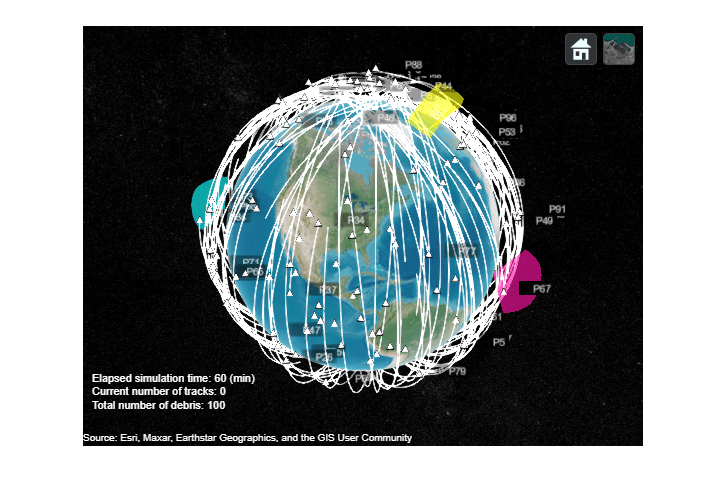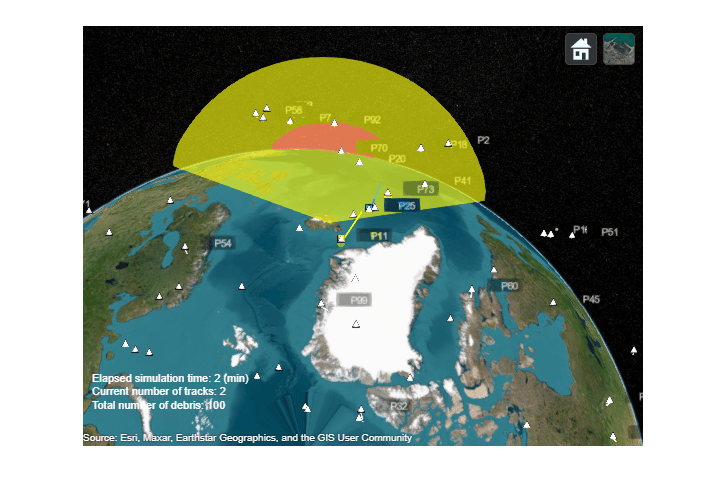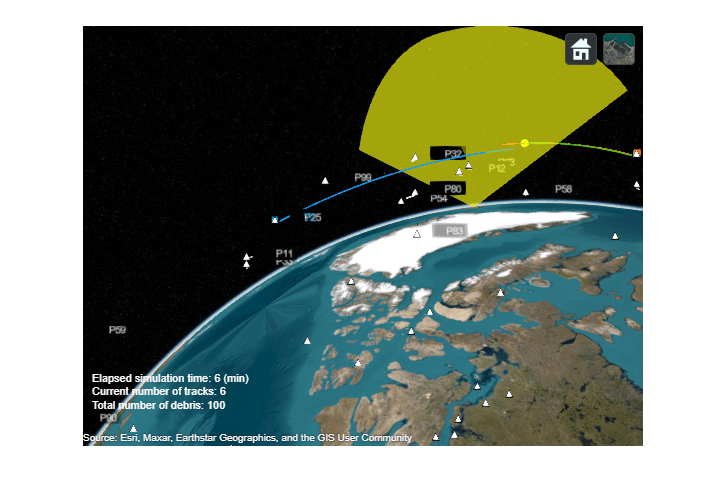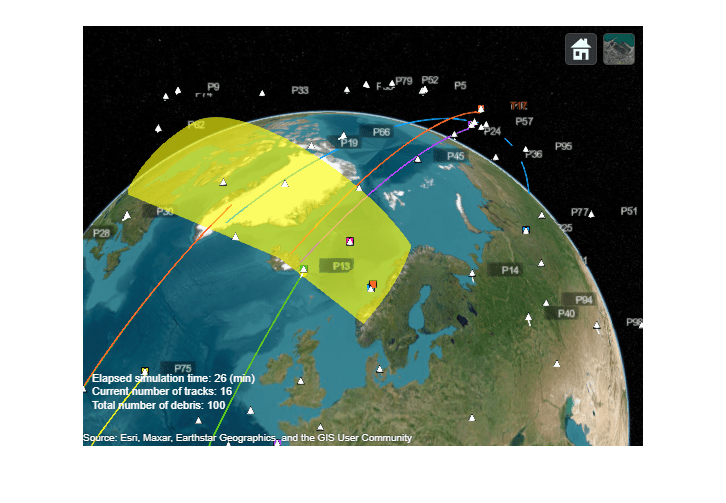Track Space Debris Using a Keplerian Motion Model

This example shows how to model earth-centric trajectories using custom motion models within trackingScenario, how to configure a fusionRadarSensor in monostatic mode to generate synthetic detections of space debris, and how to setup a multi-object tracker to track the simulated targets.

Space debris scenario

There are more than 30,000 large debris objects (with diameter larger than 10cm) and more than 1 million smaller debris objects in Low Earth Orbit (LEO) . This debris can be dangerous for human activities in space, damage operational satellites, and force time sensitive and costly avoidance maneuvers. As space activity increases, reducing and monitoring the space debris becomes crucial.

You can use Sensor Fusion and Tracking Toolbox™ to model the debris trajectories, generate synthetic radar detections of this debris, and obtain position and velocity estimates of each object.

First, create a tracking scenario and set the random seed for repeatable results.

s = rng;
rng(2020);
'StopTime', 3600, 'UpdateRate', 0.1);

You use the Earth-Centered-Earth-Fixed (ECEF) reference frame. The origin of this frame is at the center of the Earth and the Z axis points toward the north pole. The X axis points towards the intersection of the equator and the Greenwich meridian. The Y axis completes the right-handed system. Platform positions and velocities are defined using Cartesian coordinates in this frame.

Define debris motion model

The helperMotionTrajectory class used in this example defines debris object trajectories using a custom motion model function.

Trajectories of space objects rotating around the Earth can be approximated with a Keplerian model, which assumes that Earth is a point-mass body and the objects orbiting around the earth have negligible masses. Higher order effects in Earth gravitational field and environmental disturbances are not accounted for. Since the equation of motion is expressed in ECEF frame which is a non-inertial reference frame, the Coriolis and centripetal forces are accounted for.

The ECEF debris object acceleration vector is

$\stackrel{\to }{\mathit{a}}=\frac{-\mu }{{\mathit{r}}^{3}}\text{\hspace{0.17em}}\stackrel{\to }{\mathit{r}}-2\stackrel{\to }{\Omega }×\frac{\mathrm{d}}{\mathrm{d}\mathit{t}}\stackrel{\to }{\mathit{r}}-\stackrel{\to }{\Omega }×\left(\stackrel{\to }{\Omega }×\stackrel{\to }{\mathit{r}}\right)$,

where $\mu$ is the standard gravitational parameter of the Earth, $\stackrel{\to }{\mathit{r}}$ is the ECEF debris object position vector, $\mathit{r}$ is the norm of the position vector, and $\stackrel{\to }{\Omega }$is the Earth rotation vector.

The function keplerorbit provided below uses a 4th order Runge-Kutta numerical integration of this equation to propagate the position and velocity in time.

First, we create initial positions and velocities for the space debris objects. This is done by obtaining the traditional orbital elements (semi-major axis, eccentricity, inclination, longitude of the ascending node, argument of periapsis, and true anomaly angles) of these objects from random distributions. Then convert these orbital elements to position and velocity vectors by using the supporting function oe2rv.

% Generate a population of debris
numDebris = 100;

range = 7e6 + 1e5*randn(numDebris,1);
ecc = 0.015 + 0.005*randn(numDebris,1);
inc = 80 + 10*rand(numDebris,1);
lan = 360*rand(numDebris,1);
w = 360*rand(numDebris,1);
nu = 360*rand(numDebris,1);

% Convert to initial position and velocity
for i = 1:numDebris
[r,v] = oe2rv(range(i),ecc(i),inc(i),lan(i),w(i),nu(i));
data(i).InitialPosition = r; %#ok<SAGROW>
data(i).InitialVelocity = v; %#ok<SAGROW>
end

% Create platforms and assign them trajectories using the keplerorbit motion model
for i=1:numDebris
debris(i) = platform(scene); %#ok<SAGROW>
debris(i).Trajectory = helperMotionTrajectory(@keplerorbit,...
'SampleRate',0.1,... % integration step 10sec
'Position',data(i).InitialPosition,...
'Velocity',data(i).InitialVelocity); %#ok<SAGROW>
end

In this example, we define four antipodal stations with fan-shaped radar beams looking into space. The fans cut through the orbits of debris objects to maximize the number of object detections. A pair of stations are located in the Pacific ocean and in the Atlantic ocean, whereas a second pair of surveillance stations are located near the poles. Having four dispersed radars allows for the re-detection of space debris to correct their position estimates and also acquiring new debris detections.

% Create a space surveillance station in the Pacific ocean
station1 = platform(scene,'Position',[10 180 0]);

% Create a second surveillance station in the Atlantic ocean
station2 = platform(scene,'Position',[0 -20 0]);

% Near the North Pole, create a third surveillance station in Iceland
station3 = platform(scene,'Position',[65 -20 0]);

% Create a fourth surveillance station near the south pole
station4 = platform(scene,'Position',[-90 0 0]);

Each station is equipped with a radar modeled with a fusionRadarSensor object. In order to detect debris objects in the LEO range, the radar has the following requirements:

• Detecting a 10 dBsm object up to 2000 km away

• Resolving objects horizontally and vertically with a precision of 100 m at 2000 km range

• Having a fan-shaped field of view of 120 degrees in azimuth and 30 degrees in elevation

• Looking up into space based on its geo-location

% Create fan-shaped monostatic radars to monitor space debris objects
'UpdateRate',0.1,... 10 sec
'ScanMode','No scanning',...
'MountingAngles',[0 90 0],... look up
'FieldOfView',[120;30],... degrees
'ReferenceRange',2e6,... m
'RangeLimits', [0 2e6],...
'ReferenceRCS', 10,... dBsm
'HasFalseAlarms',false,...
'HasElevation',true,...
'AzimuthResolution',0.01,... degrees
'ElevationResolution',0.01,... degrees
'RangeResolution',100,... m
'HasINS',true,...
'DetectionCoordinates','Scenario');

Visualize the ground truth with trackingGlobeViewer

You use trackingGlobeViewer to visualize all the elements defined in the tracking scenario: individual debris objects and their trajectories, radar fans, radar detections, and tracks.

f = uifigure;
viewer = trackingGlobeViewer(f, 'Basemap','satellite','ShowDroppedTracks',false);
% Add info box on top of the globe viewer
infotext = simulationInfoText(0,0,0);
infobox = uilabel(f,'Text',infotext,'FontColor',[1 1 1],'FontSize',11,...
'Position',[10 20 300 70],'Visible','on');

% Show radar beams on the globe
covcon = coverageConfig(scene);
plotCoverage(viewer,covcon, 'ECEF');

infobox.Text = simulationInfoText(scene.SimulationTime, 0, numDebris);
plotPlatform(viewer, debris, 'ECEF');
end

%Take a snapshot of the scenario
snapshot(viewer);On the virtual globe, you can see the space debris represented by white dots with individual trailing trajectories shown by white lines. Most of the generated debris objects are on orbits with high inclination angles close to 80 deg.

The trajectories are plotted in ECEF coordinates, and therefore the entire trajectory rotates towards the west due to Earth rotation. After several orbit periods, all space debris pass through the surveillance beams of the radars.

Simulate synthetic detections and track space debris

The sensor models use the ground truth to generate synthetic detections. Call the detect method on the tracking scenario to obtain all the detections in the scene.

A multi-object tracker trackerJPDA is used to create new tracks, associate detections to existing tracks, estimate their state, and delete divergent tracks. Setting the property HasDetectableTrackIDsInput to true allows the tracker to accept an input that indicates whether a tracked object is detectable in the surveillance region. This is important for not penalizing tracks that are propagated outside of the radar surveillance areas. The utility function isDetectable calculates which tracks are detectable at each simulation step.

Additionally, a utility function deleteBadTracks is used to delete divergent tracks faster.

% Define Tracker
tracker = trackerJPDA('FilterInitializationFcn',@initKeplerUKF,...
'HasDetectableTrackIDsInput',true,...
'ClutterDensity',1e-20,...
'AssignmentThreshold',1e3,...
'DeletionThreshold',[7 10]);

% Reset scenario, seed, and globe display
restart(scene);
scene.StopTime = 1800; % 30 min
clear(viewer);
% Reduce history depth for debris
viewer.PlatformHistoryDepth = 2;
% Show 10-sigma covariance ellipses for better visibility
viewer.NumCovarianceSigma = 10;
plotCoverage(viewer,covcon, 'ECEF');

% Initialize tracks
confTracks = objectTrack.empty(0,1);
plotPlatform(viewer, debris);
time = scene.SimulationTime;

% Generate detections
detections = detect(scene);
plotDetection(viewer, detections, 'ECEF');

% Generate and update tracks
detectableInput = isDetectable(tracker,time, covcon);
if isLocked(tracker) || ~isempty(detections)
[confTracks, ~, allTracks,info] = tracker(detections,time,detectableInput);
plotTrack(viewer, confTracks, 'ECEF');
end

infobox.Text = simulationInfoText(time, numel(confTracks), numDebris);
% Move camera during simulation and take snapshots
switch time
case 100
campos(viewer,[90 150 5e6]);
camorient(viewer,[0 -65 345]);
drawnow
im1 = snapshot(viewer);
case 270
campos(viewer,[60 -120 2.6e6]);
camorient(viewer, [20 -45 20]);
drawnow
case 380
campos(viewer,[60 -120 2.6e6]);
camorient(viewer, [20 -45 20]);
drawnow
im2 = snapshot(viewer);
case 400
% reset
campos(viewer,[17.3 -67.2 2.400e7]);
camorient(viewer, [360 -90 0]);
drawnow
case 1500
campos(viewer,[54 2.3 6.09e6]);
camorient(viewer, [0 -73 348]);
drawnow
case 1560
im3 = snapshot(viewer);
drawnow
end
end

% Restore random seed
rng(s);
imshow(im1);On the first snapshot, you can see an object being tracked as track T1 in yellow. This object was only detected twice, which was not enough to reduce the uncertainty of the track. Therefore, the size of its covariance ellipse is relatively large. You can also observe another track T2 in blue, which is detected by the sensor several times. As a result, its corresponding covariance ellipse is much smaller since more detections were used to correct the state estimate.

imshow(im2);A few minutes later, as seen on the snapshot above, T1 was deleted because the uncertainty of the track has grown too large without detections. On the other hand, the second track T2 survived due to the additional detections.

imshow(im3)In the screenshot above, you can see that track T15 (in light blue) is about to enter the radar surveillance area. Track T11 (in orange) was just updated with a detection, which reduced the uncertainty of its estimated position and velocity. With radar station configuration, after 30 minutes of surveillance, 18 tracks were initialized and confirmed out of the 100 debris objects. If you increase the simulation time, the radars will cover 360 degrees in space and eventually more debris can be tracked. Different radar station locations and configurations could be explored to increase the number of tracked objects.

Summary

In this example you have learned how to specify your own motion model to move platforms in a tracking scenario and how to use them to setup a tracker. This enables you to apply sensor fusion and tracking techniques offered in this toolbox to a wider range of applications, such as the problem of modelling and tracking space debris in an Earth-Centered-Earth-Fixed coordinate frame as shown in this example.

Supporting functions

The motion model used in this example is presented below. The state is the ECEF positions and velocities of the object [x; vx; y; vy; z; vz].

function state = keplerorbit(state,dt)
% keplerorbit performs numerical integration to predict the state of
% keplerian bodies. The state is [x;vx;y;vy;z;vz]

% Runge-Kutta 4 integration method:
k1 = kepler(state);
k2 = kepler(state + dt*k1/2);
k3 = kepler(state + dt*k2/2);
k4 = kepler(state + dt*k3);

state = state + dt*(k1+2*k2+2*k3+k4)/6;

function dstate=kepler(state)
x =state(1,:);
vx = state(2,:);
y=state(3,:);
vy = state(4,:);
z=state(5,:);
vz = state(6,:);

mu = 398600.4405*1e9; % m^3 s^-2

r = norm([x y z]);
g = mu/r^2;

% Coordinates are in a non-intertial frame, account for Coriolis
% and centripetal acceleration
ax = -g*x/r + 2*omega*vy + omega^2*x;
ay = -g*y/r - 2*omega*vx + omega^2*y;
az = -g*z/r;
dstate = [vx;ax;vy;ay;vz;az];
end
end

initKeplerUKF initializes a tracking filter with your own motion model. In this example, we use the same motion model that establishes ground truth, keplerorbit.

function filter = initKeplerUKF(detection)

% assumes radar returns [x y z]
measmodel= @(x,varargin) x([1 3 5],:);
detNoise = detection.MeasurementNoise;
sigpos = 0.4;% m
sigvel = 0.4;% m/s^2
meas = detection.Measurement;
initState = [meas(1); 0; meas(2); 0; meas(3);0];
filter = trackingUKF(@keplerorbit,measmodel,initState,...
'StateCovariance', diag(repmat([10, 10000].^2,1,3)),...
'ProcessNoise',diag(repmat([sigpos, sigvel].^2,1,3)),...
'MeasurementNoise',detNoise);

end

oe2rv converts a set of 6 traditional orbital elements to position and velocity vectors.

function [r,v] = oe2rv(a,e,i,lan,w,nu)
% Reference: Bate, Mueller & White, Fundamentals of Astrodynamics Sec 2.5

mu = 398600.4405*1e9; % m^3 s^-2

% Express r and v in perifocal system
cnu = cosd(nu);
snu = sind(nu);
p = a*(1 - e^2);
r = p/(1 + e*cnu);
r_peri = [r*cnu ; r*snu ; 0];
v_peri = sqrt(mu/p)*[-snu ; e + cnu ; 0];

% Tranform into Geocentric Equatorial frame
clan = cosd(lan);
slan = sind(lan);
cw = cosd(w);
sw = sind(w);
ci = cosd(i);
si = sind(i);
R = [ clan*cw-slan*sw*ci  ,  -clan*sw-slan*cw*ci   ,    slan*si; ...
slan*cw+clan*sw*ci  ,   -slan*sw+clan*cw*ci  ,   -clan*si; ...
sw*si                  ,   cw*si                   ,   ci];
r = R*r_peri;
v = R*v_peri;
end

isDetectable is used in the example to determine which tracks are detectable at a given time.

function detectInput = isDetectable(tracker,time,covcon)

if ~isLocked(tracker)
detectInput = zeros(0,1,'uint32');
return
end
tracks = tracker.predictTracksToTime('all',time);
if isempty(tracks)
detectInput = zeros(0,1,'uint32');
else
alltrackid = [tracks.TrackID];
isDetectable = zeros(numel(tracks),numel(covcon),'logical');
for i = 1:numel(tracks)
track = tracks(i);
pos_scene = track.State([1 3 5]);
for j=1:numel(covcon)
config = covcon(j);
% rotate position to sensor frame:
d_scene = pos_scene(:) - config.Position(:);
scene2sens = rotmat(config.Orientation,'frame');
d_sens = scene2sens*d_scene(:);
[az,el] = cart2sph(d_sens(1),d_sens(2),d_sens(3));
isDetectable(i,j) = true;
else
isDetectable(i,j) = false;
end
end
end

detectInput = alltrackid(any(isDetectable,2))';
end
end

deleteBadTracks is used to remove tracks that obviously diverged. Specifically, diverged tracks in this example are tracks whose current position has fallen on the surface of the earth and tracks whose covariance has become too large.

% remove divergent tracks:
%   - tracks with covariance > 4*1e8 (20 km standard deviation)
%   - tracks with estimated position outside of LEO bounds
n = numel(tracks);
toDelete = zeros(1,n,'logical');
for i=1:numel(tracks)
[pos, cov] = getTrackPositions(tracks(i),[ 1 0 0 0 0 0 ; 0 0 1 0 0 0; 0 0 0 0 1 0]);
if norm(pos) < 6500*1e3 || norm(pos) > 8500*1e3 || max(cov,[],'all') > 4*1e8
deleteTrack(tracker,tracks(i).TrackID);
toDelete(i) =true;
end
end
tracks(toDelete) = [];
end

simulationInfoText is used to display the simulation time, the number of debris, and the current number of tracks in the scenario.

function text = simulationInfoText(time,numTracks, numDebris)
text = vertcat(string(['Elapsed simulation time: ' num2str(round(time/60)) ' (min)']),...
string(['Current number of tracks: ' num2str(numTracks)]),...
string(['Total number of debris: ' num2str(numDebris)]));
drawnow limitrate
end